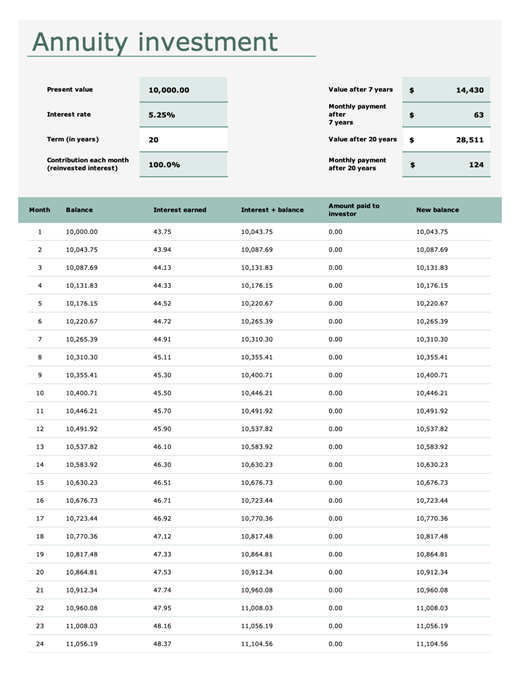# Annuity investment calculator

This worksheet template calculates the monthly value of an annuity investment. Simply enter the present value, interest rate, term, and contribution of reinvested interest each month, and interest and balances are calculated automatically. Instructions are provided for each of the fill-in values.

Excel